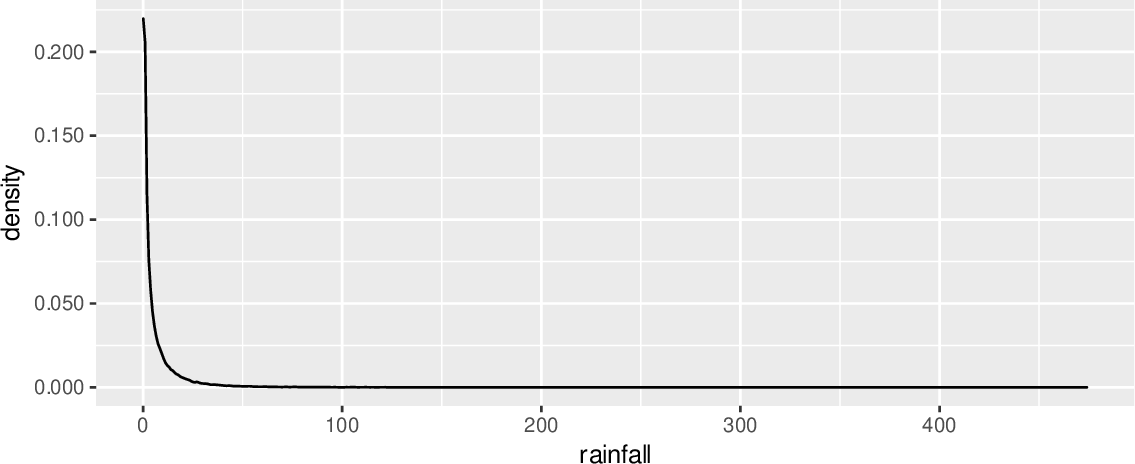Data Science Desktop Survival Guide by Graham WilliamsDesktop Survival Project Home Preface Data Science Introducing R R Constructs R Tasks R Strings R Read, Write, and Create Data Template Data Exploration Data Wrangling Data Visualisation Statistics ML Template ML Scenarios ML Activities ML Applications ML Algorithms Cluster Analysis Decision Trees Computer Vision Graph Data Privacy Literate Data Science Coding with Style Resources Bibliography Index

## Line Chart Skewed Distributions

20200823ds %>%   filter(rainfall != 0) %>%   ggplot(aes(x=rainfall)) +   geom_density() +   scale_y_continuous(labels=comma) +   theme(legend.position="none") The plot informs us about the skewed nature of the amount of rainfall recorded for any one day, but we lose a lot of resolution at the low end. Note that we use a subset of the dataset to include only those observations of rainfall (i.e., where the rainfall is non-zero). Otherwise a warning will note many rows contain non-finite values in calculating the density statistic.